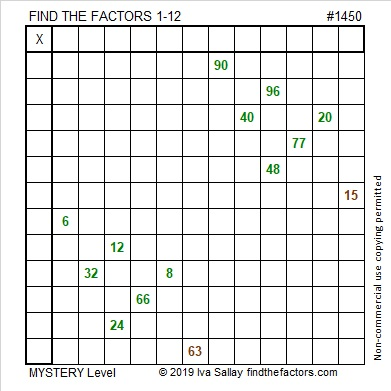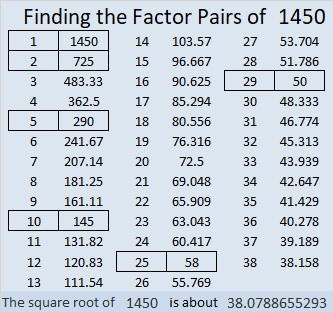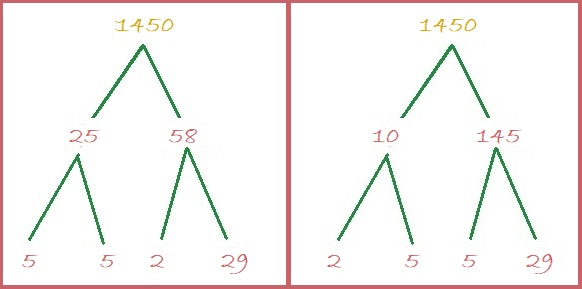# 1450 A Pair of Factor Trees

On today’s puzzle, there are two small Christmas trees. Will two smaller trees on the puzzle be easier to solve than one big one? You’ll have to try it to know!Every puzzle has a puzzle number to distinguish it from the others. Here are some facts about this puzzle number, 1450:

• 1450 is a composite number.
• Prime factorization: 1450 = 2 × 5 × 5 × 29, which can be written 1450 = 2 × 5² × 29
• 1450 has at least one exponent greater than 1 in its prime factorization so √1450 can be simplified. Taking the factor pair from the factor pair table below with the largest square number factor, we get √1450 = (√25)(√58) = 5√58
• The exponents in the prime factorization are 1, 2, and 1. Adding one to each exponent and multiplying we get (1 + 1)(2 + 1)(1 + 1) = 2 × 3 × 2 = 12. Therefore 1450 has exactly 12 factors.
• The factors of 1450 are outlined with their factor pair partners in the graphic below.In case you are looking for factor trees for 1450, here are two different ones:1450 is the hypotenuse of SEVEN Pythagorean triples:
170-1440-1450 which is 10 times (17-144-145)
240-1430-1450 which is 10 times (24-143-145)
406-1392-1450 which is (7-24-25) times 58
666-1288-1450 which is 2 times (333-644-725)
728-1254-1450 which is 2 times (364-627-725)
870-1160-1450 which is (3-4-5) times 290
1000-1050-1450 which is (20-21-29) times 50

This site uses Akismet to reduce spam. Learn how your comment data is processed.